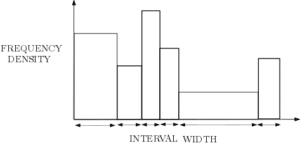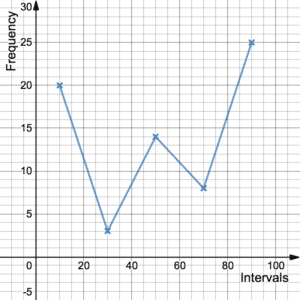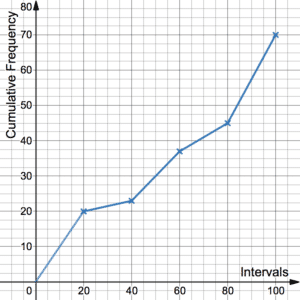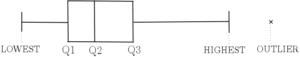# Data Presentation

Data presentation is the way in which data is displayed. Indeed, there are various ways in which this can be done. The best method to choose will depend on the type of data you have, how you want it to look and what you would like to see. More often than not it will be evident from the exam question which method you should use. The following methods provide ways to display univariate data (or a list of single data points that may or may not be grouped).

## HistogramsHistograms are a useful method of data presentation. Students often get confused between histograms and bar charts. However, histograms and bar charts are different in a number of ways. Firstly, histograms are used to present continuous data whereas bar charts are used to display discrete data. Secondly, and more importantly, histograms are not plotted against frequency, they are plotted against frequency density. This means that the frequency is represented in the area of the bar.

## Frequency PolygonsFrequency polygons are a common form of chart used in data presentation. Frequencies are plotted on the graph as points (or crosses etc.) and joined up by straight lines. They are called frequency polygons because polygons are shapes where the vertices are joined up by straight lines. However, the first and final points are not joined up so it should be thought of as more of an open shape.

See more on Frequency Polygons.

## Cumulative Frequency DiagramsCumulative is the adjective for accumulate. It means to add up successively. It follows that cumulative frequency is where the frequencies are added up at each step.
The lower quartile, the median and the upper quartile can be identified from a cumulative frequency diagram.

See more on Cumulative Frequency Diagrams.

## Box PlotsIn order to draw a box plot, you need to know the lower quartile, the median and the upper quartile. As opposed to a cumulative frequency diagram, these values are identified horizontally. Box plots may also have outliers. These are usually identified by a cross outside the main box plot either to the left or right of the box plot or both. See more about outliers.

See more on Box Plots.

Link to Probability Distributions – TBC

Click here to see an example of real data. This chart shows three frequency polygons related to climate change according to three different mathematical models. The grey area represents uncertainty.